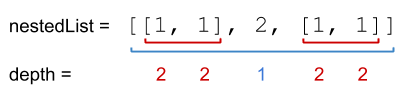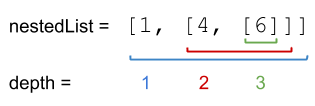## 339. Nested List Weight Sum

You are given a nested list of integers `nestedList`. Each element is either an integer or a list whose elements may also be integers or other lists.

The depth of an integer is the number of lists that it is inside of. For example, the nested list `[1,[2,2],[,2],1]` has each integer's value set to its depth.

Return the sum of each integer in `nestedList` multiplied by its depth.

Example 1:```Input: nestedList = [[1,1],2,[1,1]]
Output: 10
Explanation: Four 1's at depth 2, one 2 at depth 1. 1*2 + 1*2 + 2*1 + 1*2 + 1*2 = 10.
```

Example 2:```Input: nestedList = [1,[4,]]
Output: 27
Explanation: One 1 at depth 1, one 4 at depth 2, and one 6 at depth 3. 1*1 + 4*2 + 6*3 = 27.```

Example 3:

```Input: nestedList = 
Output: 0
```

Constraints:

• `1 <= nestedList.length <= 50`
• The values of the integers in the nested list is in the range `[-100, 100]`.
• The maximum depth of any integer is less than or equal to `50`.

## Rust Solution

``````struct Solution;
use rustgym_util::*;

impl Solution {
fn depth_sum_r(nested_list: &NestedInteger, level: i32) -> i32 {
match nested_list {
NestedInteger::Int(x) => level * x,
NestedInteger::List(v) => v
.iter()
.fold(0, |acc, x| acc + Self::depth_sum_r(x, level + 1)),
}
}
fn depth_sum(nested_list: Vec<NestedInteger>) -> i32 {
nested_list
.iter()
.fold(0, |acc, x| acc + Self::depth_sum_r(x, 1))
}
}

#[test]
fn test() {
let list = vec![
nested_integer!("[1,1]"),
nested_integer!("2"),
nested_integer!("[1,1]"),
];
assert_eq!(Solution::depth_sum(list), 10);
let list = vec![nested_integer!("1"), nested_integer!("[4,]")];
assert_eq!(Solution::depth_sum(list), 27);
}
``````

Having problems with this solution? Click here to submit an issue on github.